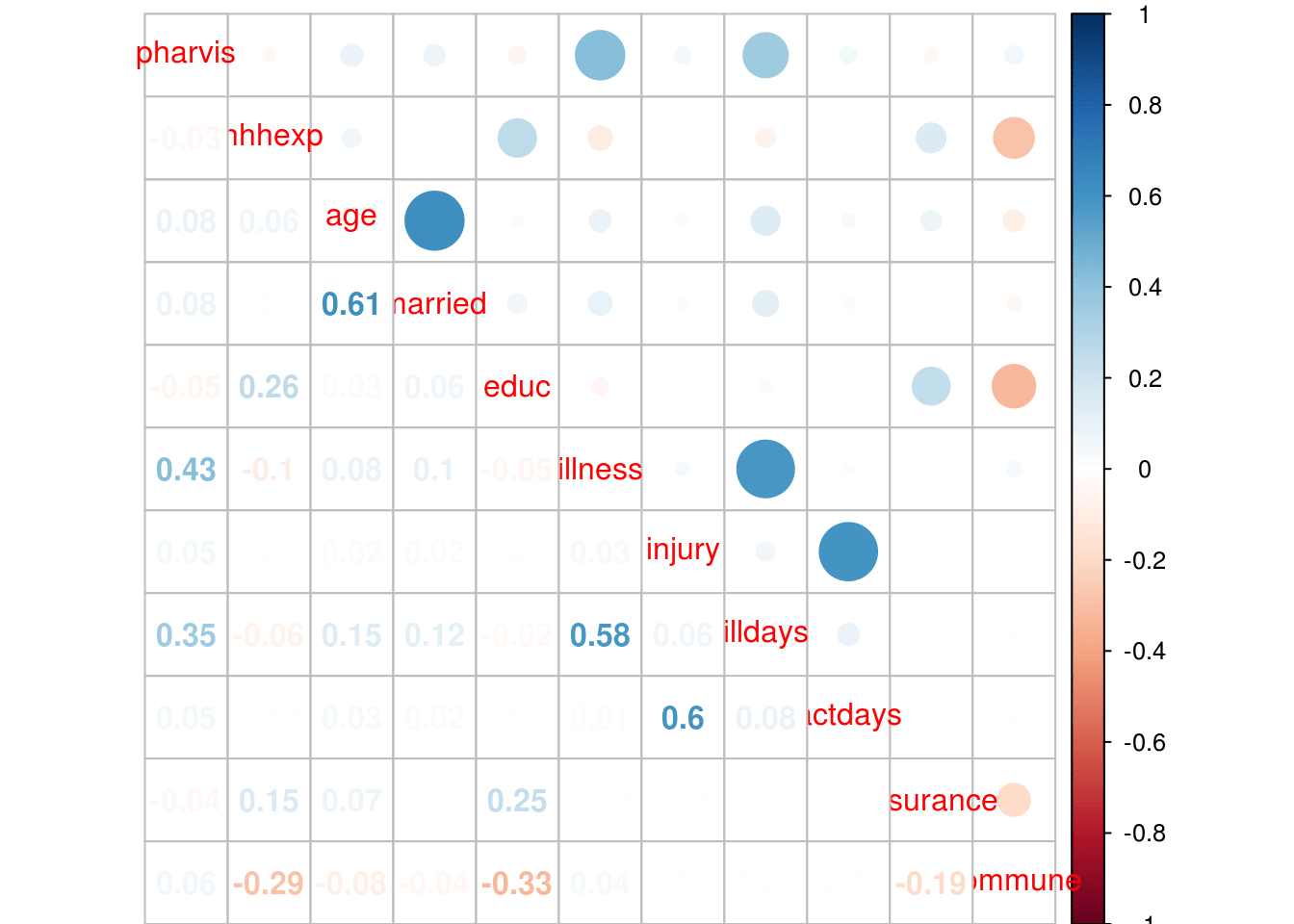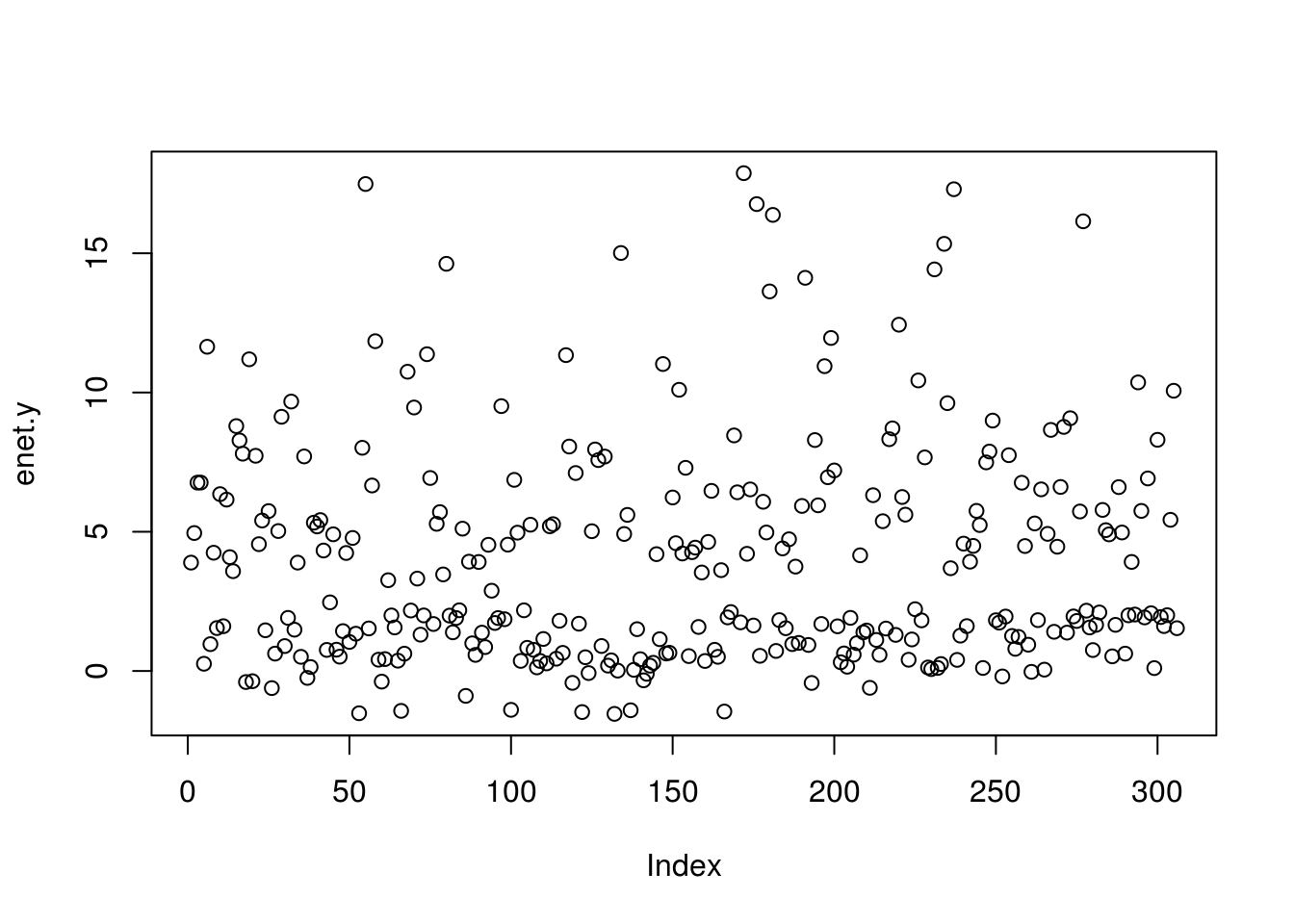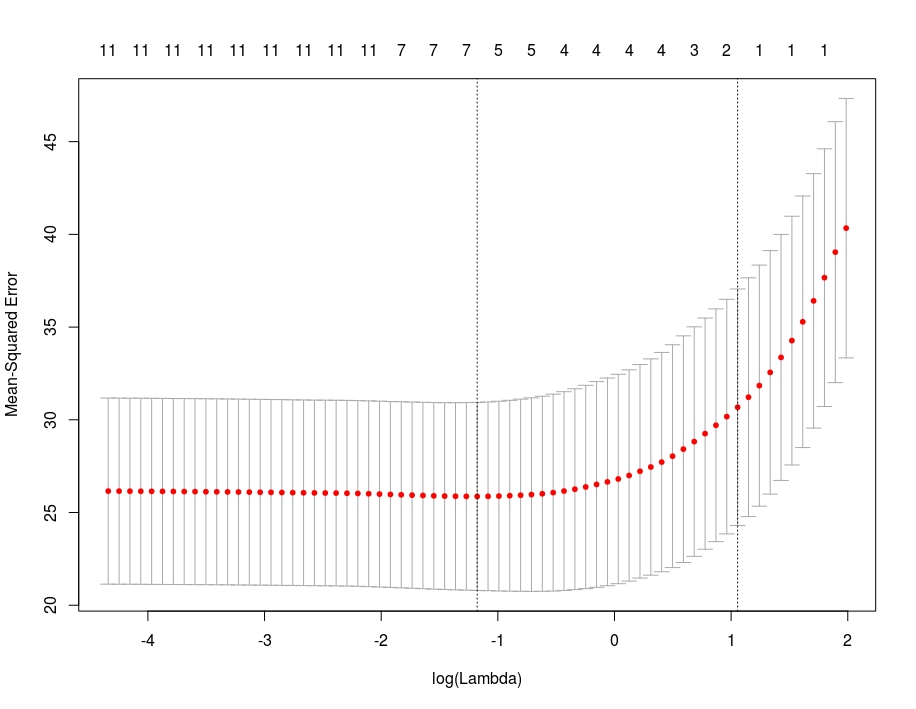# Elastic Net Regression in R

Elastic net is a combination of ridge and lasso regression. What is most unusual about elastic net is that it has two tuning parameters (alpha and lambda) while lasso and ridge regression only has 1.

In this post, we will go through an example of the use of elastic net using the“VietnamI” dataset from the “Ecdat” package. Our goal is to predict how many days a person is ill based on the other variables in the dataset. Below is some initial code for our analysis

``library(Ecdat);library(corrplot);library(caret);library(glmnet)``
``````data("VietNamI")
str(VietNamI)``````
``````## 'data.frame':    27765 obs. of  12 variables:
##  \$ pharvis  : num  0 0 0 1 1 0 0 0 2 3 ...
##  \$ lnhhexp  : num  2.73 2.74 2.27 2.39 3.11 ...
##  \$ age      : num  3.76 2.94 2.56 3.64 3.3 ...
##  \$ sex      : Factor w/ 2 levels "female","male": 2 1 2 1 2 2 1 2 1 2 ...
##  \$ married  : num  1 0 0 1 1 1 1 0 1 1 ...
##  \$ educ     : num  2 0 4 3 3 9 2 5 2 0 ...
##  \$ illness  : num  1 1 0 1 1 0 0 0 2 1 ...
##  \$ injury   : num  0 0 0 0 0 0 0 0 0 0 ...
##  \$ illdays  : num  7 4 0 3 10 0 0 0 4 7 ...
##  \$ actdays  : num  0 0 0 0 0 0 0 0 0 0 ...
##  \$ insurance: num  0 0 1 1 0 1 1 1 0 0 ...
##  \$ commune  : num  192 167 76 123 148 20 40 57 49 170 ...
##  - attr(*, "na.action")=Class 'omit'  Named int 27734
##   .. ..- attr(*, "names")= chr "27734"``````

We need to check the correlations among the variables. We need to exclude the “sex” variable as it is categorical. The code is below.

``````p.cor<-cor(VietNamI[,-4])
corrplot.mixed(p.cor)``````No major problems with correlations. Next, we set up our training and testing datasets. We need to remove the variable “commune” because it adds no value to our results. In addition, to reduce the computational time we will only use the first 1000 rows from the data set.

``````VietNamI\$commune<-NULL
VietNamI_reduced<-VietNamI[1:1000,]
ind<-sample(2,nrow(VietNamI_reduced),replace=T,prob = c(0.7,0.3))
train<-VietNamI_reduced[ind==1,]
test<-VietNamI_reduced[ind==2,]``````

We need to create a grid that will allow us to investigate different models with different combinations of alpha and lambda. This is done using the “expand.grid” function. In combination with the “seq” function below is the code

``grid<-expand.grid(.alpha=seq(0,1,by=.5),.lambda=seq(0,0.2,by=.1))``

We also need to set the resampling method, which allows us to assess the validity of our model. This is done using the “trainControl” function” from the “caret” package. In the code below “LOOCV” stands for “leave one out cross-validation”.

``control<-trainControl(method = "LOOCV")``

We are no ready to develop our model. The code is mostly self-explanatory. This initial model will help us to determine the appropriate values for the alpha and lambda parameters

``````enet.train<-train(illdays~.,train,method="glmnet",trControl=control,tuneGrid=grid)
enet.train``````
``````## glmnet
##
## 694 samples
##  10 predictors
##
## No pre-processing
## Resampling: Leave-One-Out Cross-Validation
## Summary of sample sizes: 693, 693, 693, 693, 693, 693, ...
## Resampling results across tuning parameters:
##
##   alpha  lambda  RMSE      Rsquared
##   0.0    0.0     5.229759  0.2968354
##   0.0    0.1     5.229759  0.2968354
##   0.0    0.2     5.229759  0.2968354
##   0.5    0.0     5.243919  0.2954226
##   0.5    0.1     5.225067  0.2985989
##   0.5    0.2     5.200415  0.3038821
##   1.0    0.0     5.244020  0.2954519
##   1.0    0.1     5.203973  0.3033173
##   1.0    0.2     5.182120  0.3083819
##
## RMSE was used to select the optimal model using  the smallest value.
## The final values used for the model were alpha = 1 and lambda = 0.2.``````

The output list all the possible alpha and lambda values that we set in the “grid” variable. It even tells us which combination was the best. For our purposes, the alpha will be .5 and the lambda .2. The r-square is also included.

We will set our model and run it on the test set. We have to convert the “sex” variable to a dummy variable for the “glmnet” function. We next have to make matrices for the predictor variables and a for our outcome variable “illdays”

``````train\$sex<-model.matrix( ~ sex - 1, data=train ) #convert to dummy variable
test\$sex<-model.matrix( ~ sex - 1, data=test )
predictor_variables<-as.matrix(train[,-9])
days_ill<-as.matrix(train\$illdays)
enet<-glmnet(predictor_variables,days_ill,family = "gaussian",alpha = 0.5,lambda = .2)``````

We can now look at specific coefficient by using the “coef” function.

``````enet.coef<-coef(enet,lambda=.2,alpha=.5,exact=T)
enet.coef``````
``````## 12 x 1 sparse Matrix of class "dgCMatrix"
##                         s0
## (Intercept)   -1.304263895
## pharvis        0.532353361
## lnhhexp       -0.064754000
## age            0.760864404
## sex.sexfemale  0.029612290
## sex.sexmale   -0.002617404
## married        0.318639271
## educ           .
## illness        3.103047473
## injury         .
## actdays        0.314851347
## insurance      .``````

You can see for yourself that several variables were removed from the model. Medical expenses (lnhhexp), sex, education, injury, and insurance do not play a role in the number of days ill for an individual in Vietnam.

With our model developed. We now can test it using the predict function. However, we first need to convert our test dataframe into a matrix and remove the outcome variable from it

``````test.matrix<-as.matrix(test[,-9])
enet.y<-predict(enet, newx = test.matrix, type = "response", lambda=.2,alpha=.5)``````

Let’s plot our results

``plot(enet.y)``This does not look good. Let’s check the mean squared error

``````enet.resid<-enet.y-test\$illdays
mean(enet.resid^2)``````
``##  20.18134``

We will now do a cross-validation of our model. We need to set the seed and then use the “cv.glmnet” to develop the cross-validated model. We can see the model by plotting it.

``````set.seed(317)
enet.cv<-cv.glmnet(predictor_variables,days_ill,alpha=.5)
plot(enet.cv)``````You can see that as the number of features are reduce (see the numbers on the top of the plot) the MSE increases (y-axis). In addition, as the lambda increases, there is also an increase in the error but only when the number of variables is reduced as well.

The dotted vertical lines in the plot represent the minimum MSE for a set lambda (on the left) and the one standard error from the minimum (on the right). You can extract these two lambda values using the code below.

``enet.cv\$lambda.min``
``##  `0.3082347`
``enet.cv\$lambda.1se``
``##  `2.874607`

We can see the coefficients for a lambda that is one standard error away by using the code below. This will give us an alternative idea for what to set the model parameters to when we want to predict.

``coef(enet.cv,s="lambda.1se")``
``````## 12 x 1 sparse Matrix of class "dgCMatrix"
##                      1
## (Intercept)   2.34116947
## pharvis       0.003710399
## lnhhexp       .
## age           .
## sex.sexfemale .
## sex.sexmale   .
## married       .
## educ          .
## illness       1.817479480
## injury        .
## actdays       .
## insurance     .``````

Using the one standard error lambda we lose most of our features. We can now see if the model improves by rerunning it with this information.

``````enet.y.cv<-predict(enet.cv,newx = test.matrix,type='response',lambda="lambda.1se", alpha = .5)
enet.cv.resid<-enet.y.cv-test\$illdays
mean(enet.cv.resid^2)``````
``##  25.47966``

A small improvement.  Our model is a mess but this post served as an example of how to conduct an analysis using elastic net regression.